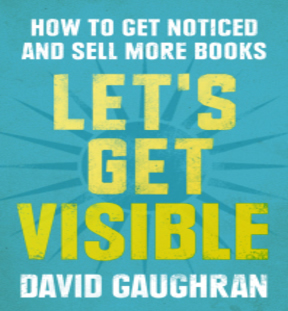# Sales Ranking ChartHere’s an interesting chart I ran across in David Gaughran’s book, “Let’s Get Visible.” If you haven’t read his book, I highly recommend it.  The chart serves as a guideline for estimating how many sales are required for an eBook to make it on the Best Seller List in the Amazon US store.

### Rank to Sales Estimator

#1 to #5 = 3,500+ books a day (sometimes a lot more)

#5 to #10 = 2,000 to 3,500 per day

#10 to #20 = 1,100 to 2,000

#20 to #65 = 850-1,100

#80 = 850

#90 = 750

#275 = 325

#500 = 200

#1000 = 100

#2000 = 50-55

#3000 = 40-45

#4000 = 30

#5000 = 20-25

#7500 = 16

#12000 = 10

#25000 = 5

#32000 = 3

#42500 = 2

#70000 to #100,0000 = 1

#1000,000+ = less than 1 per day